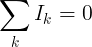Kirchhoff circuit laws - Electrical Engineering Gate

# Electrical Engineering Gate

Online Electrical Engineering Study Site

## Thursday, October 16, 2014

Kirchhoff's circuit laws are two equalities that deal with the current and potential difference (commonly known as voltage) in the lumped element model of electrical circuits. They were first described in 1845 by German physicist Gustav Kirchhoff .This generalized the work of Georg Ohm and preceded the work of Maxwell. Widely used in electrical engineering, they are also called Kirchhoff's rules or simply Kirchhoff's laws
Both of Kirchhoff's laws can be understood as corollaries of the Maxwell equations in the low-frequency limit. They are accurate for DC circuits, and for AC circuits at frequencies where the wavelengths of electromagnetic radiation are very large compared to the circuits

## (Kirchhoff's Current Law (KCL

This law is also called Kirchhoff's first lawKirchhoff's point rule, or Kirchhoff's junction rule
.This is Kirchhoff's first law
The sum of all currents that enter an electrical circuit junction is 0. When the currents enter the junction have positive sign and the current that leave the junction have negative signAnother way to look at this law is that the sum of currents that enter a junction is equal to the sum of currents that leave the junction

#### KCL example

I1 and I2 enter the junction
I3 leave the junction
?=I1=2A, I2=3A, I3=-1A, I4

Solution
Ik = I1+I2+I3+I= 0
I4 = -I1 - I2 - I= -2A - 3A - (-1A) = -4A
Since I4 is negative, it leaves the junction

## (Kirchhoff's Voltage Law (KVL

This law is also called Kirchhoff's second law, Kirchhoff's loop (or mesh) rule, and Kirchhoff's second rule
This is Kirchhoff's second law
The sum of all voltages or potential differences in an electrical circuit loop is 0#### KVL example

VS = 12V, VR1 = -4V, VR2 = -3V
?=VR3
Solution
Vk = V+ VR1 + VR2 + VR3 = 0
VR3 = -VS  - VR1 - VR2 = -12V+4V+3V = -5V
The voltage sign (+/-) is the direction of the potential difference